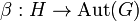# Tensor product of groups

## History

The concept of tensor product of (not necessarily abelian) groups was introduced by Loday and Brown in their paper on van Kampen's theorem.

## Definition

### Direct definition

Suppose$G$ and$H$ are (not necessarily abelian) groups with a compatible pair of actions$\alpha:G \to \operatorname{Aut}(H)$ and$\beta:H \to \operatorname{Aut}(G)$. The tensor product (sometimes called the non-abelian tensor product) of$G$ and$H$ for this pair of actions, denoted$G \otimes H$, is defined as the quotient group of the free group on the set of symbols$\{ g \otimes h \mid g \in G, h \in H \}$ by the following relations:

•$(g_1g_2) \otimes h = (g_1g_2g_1^{-1} \otimes \alpha(g_1)h)(g_1 \otimes h)$, one such relation for all$g_1,g_2 \in G, h \in H$
•$g \otimes (h_1h_2) = (g \otimes h_1)(\beta(h_1)g \otimes h_1h_2h_1^{-1})$, one such relation for all$g \in G, h_1,h_2 \in H$

If we use$\cdot$ to denote$\alpha,\beta$ and the actions by conjugation of the groups on themselves, this becomes:

•$(g_1g_2) \otimes h = ((g_1 \cdot g_2) \otimes (g_1 \cdot h))(g_1 \otimes h) \ \forall \ g_1,g_2 \in G, h \in H$
•$g \otimes (h_1h_2) = (g \otimes h_1)((h_1 \cdot g) \otimes (h_1 \cdot h_2)) \ \forall \ g \in G, h_1,h_2 \in H$

### Definition in terms of crossed pairings

Suppose$G$ and$H$ are (not necessarily abelian) groups with a compatible pair of actions$\alpha:G \to \operatorname{Aut}(H)$ and$\beta:H \to \operatorname{Aut}(G)$. The tensor product (sometimes called the non-abelian tensor product) of$G$ and$H$ for this pair of actions, denoted$G \otimes H$, is defined as the universal (initial) object among crossed pairings of$G$ and$H$ for the compatible pair of actions. Explicitly,$G \otimes H$ is a group along with a set map$s:G \times H \to G \otimes H$ such that, for any group$K$, if we consider the mapping:$\varphi \mapsto \varphi \circ s$

Then this mapping establishes a bijection from the set of group homomorphisms$G \otimes H \to K$ to the set of crossed pairings from$G \times H$ to$K$.

This latter definition is very useful in terms of showing that certain maps from the tensor product, defined on generators, are indeed well-defined homomorphisms. The idea is to show that the rule used to do the map on generators defines a crossed pairing.

## Facts

### Maps and constructions

For the statements in these facts, we will use the same notation as in the definition above:$G$ and$H$ are groups with a compatible pair of actions$\alpha:G \to \operatorname{Aut}(H)$ and$\beta:H \to \operatorname{Aut}(G)$.

Name The kind of map or construction Explicit description using named actions Explicit description using$\cdot$
Tensor product of groups is commutative up to natural isomorphism A natural isomorphism$G \otimes H \to H \otimes G$. If the isomorphism is applied twice, it gives the identity mapping.$g \otimes h \mapsto (h \otimes g)^{-1}$$g \otimes h \mapsto (h \otimes g)^{-1}$
Tensor product of groups maps to both groups Homomorphisms:$\lambda_G: G \otimes H \to G$$\lambda_H: G \otimes H \to H$$\lambda_G = g \otimes h \mapsto g(\beta(h)(g^{-1}))$$\lambda_H = g \otimes h \mapsto (\alpha(g)(h))h^{-1}$$\lambda_G = g \otimes h \mapsto g(h \cdot g^{-1})$$\lambda_H = g \otimes h \mapsto (g \cdot h)h^{-1}$
Group acts naturally on its tensor product with any group Homomorphisms$G \to \operatorname{Aut}(G \otimes H)$$H \to \operatorname{Aut}(G \otimes H)$
The action of$g_1$ on$g_2 \otimes h$ gives$(g_1g_2g_1^{-1}) \otimes (\alpha(g_1)(h))$
The action of$h_1$ on$g \otimes h_2$ gives$(\beta(h_1)(g)) \otimes h_1h_2h_1^{-1}$$g_1 \cdot (g_2 \otimes h) = (g_1 \cdot g_2) \otimes (g_1 \otimes h)$$h_1 \cdot (g \otimes h_2) = (h_1 \cdot g) \otimes (h_1 \cdot h_2)$

### Other facts

• Tensor product of groups has a crossed module structure with respect to each group: This says that the homomorphism$G \otimes H \to G$ and the group action of$G$ on$G \otimes H$ together make$G \otimes H$ a crossed module over$G$.
• Tensor product of finite groups is finite
• Tensor product of p-groups is p-group

## Particular cases

• In case that both$G,H$ are subgroups in some big group$Q$ and they normalize each other, we can take the actions on each other as action by conjugation. These actions form a compatible pair of actions, hence it makes sense to take the tensor product of the two groups.
• In case that both the groups are abelian groups and both the maps$\alpha,\beta$ are trivial maps (i.e., each group acts trivially on the other), this becomes the usual tensor product of abelian groups.
• In case that the actions of the groups on each other are trivial, but the groups are not necessarily abelian, the tensor product is the tensor product of abelian groups of the abelianizations of the two groups. In other words, with trivial actions, the tensor product cares only about the abelianizations.